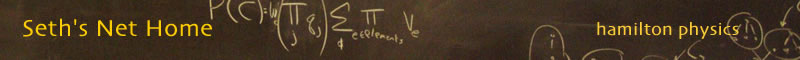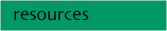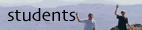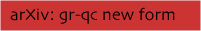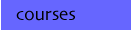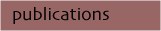##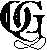What is Quantum Gravity?

Quantum gravity is the field devoted to finding the microstructure of spacetime. Is space continuous? Does spacetime geometry make sense near the initial singularity? Deep inside a black hole? These are the sort of questions a theory of quantum gravity is expected to answer. The root of our search for the theory is a exploration of the quantum foundations of spacetime. At the very least, quantum gravity ought to describe physics on the smallest possible scales - expected to be 10-35 meters. (Easy to find with dimensional analysis: Build a quantity with the dimensions of length using the speed of light, Planck's constant, and Newton's constant.) Whether quantum gravity will yield a revolutionary shift in quantum theory, general relativity, or both remains to be seen.

One path to find the theory of quantum gravity starts with general relativity as Einstein conceived it, introduces new variables, and then quantizes the resulting theory using techniques of the Hamiltonian formulation. Known as "loop Quantum Gravity" (lQG) it rests on the key elements of non-perturbative quantization and background independence. While the project is by no means complete, the kinematics are established (space!). Although the dynamics remains controversial, there has been much work on the subject...

Dirac and Arnowitt, Deser, and Misner (ADM) laid much of the groundwork for the Hamiltonian formulation when they expressed general relativity in 3+1 form, separating the three spatial dimensions from time. As a consequence of general covariance, relativity becomes entirely constrained; the equations of motion are a list of expressions which must vanish. At each "instant," the degrees of freedom are the coordinate independent part of the metric known as the "3-geometry." This observation effectively solves all but one constraint -- the Hamiltonian constraint. In the quantum theory each constraint is promoted to an operator. The physical states are those states on which the constraints vanish. The final piece of the quantization is finding solutions of the quantum Hamiltonian constraint equation, the "Wheeler-DeWitt equation."

E except for model systems, only modest progress was made in canonical quantum gravity until the late 1980's when Ashtekar discovered a new set of variables. This breakthrough allowed one to express the constraints of general relativity as polynomials in the basic variables. Even better, the variables are very similar to those used in gauge theory. The two canonical variables are an su(2) connection and a conjugate "electric field." (The original Ashtekar connection took values in a complexified su(2) algebra. Most recent work uses a real connection.) General relativity becomes a theory of three constraints: Gauss, vector, and Hamiltonian. The metric is no longer a basic variable but a derived quantity; the electric field is a "square root" of the metric. Though the metric is lost as a basic variable, these new variables enjoy a close connection with those used in gauge theories. This similarity led Rovelli and Smolin to a representation of the 3-geometry states. In this work, loops form a representation just as Wilson loops form a representation in lattice gauge theory. In general, the states of quantum gravity are intersecting, knotted loops or embedded graphs. One immediate consequence is that metric and geometric quantities are only defined along the loops.

Beginning in 1994, an understanding of quantum geometry emerged. Motivated by the need for geometric observables in the canonical approach to quantum gravity and placed on mathematically rigorous foundations, the new framework for the structure of space is an echo of an older, combinatorial definition of spacetime advocated by Penrose. Space is represented as a "spin network." From the point of lQG spin networks provide linear combinations of loops which form a basis for spatial states. One tiny piece might look like this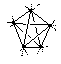Spin networks are labeled, embedded graphs. Edges carry spin excitations of geometry. These networks label a basis for states of spatial geometry. The spectra of area, volume, and angle operators are discrete. In this basis one also has many calculational methods, including a graphical calculus which enjoys close ties to knot theory and recoupling theory. So far the picture which has been uncovered is that space, on the smallest of scales, is predicted to be discrete and fabric-like in nature. Space, matter and all only exist on the edges and vertices of the spin network!

In the last few years a spacetime description

For more on spin networks, the discrete geometry, and possible observable consequences see my introduction Towards Quantum Gravity: Discrete geometry - Observable consequences (pdf). There is also a reading list for loop quantum gravity